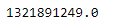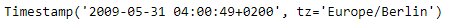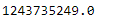# Python | Pandas Timestamp.timestamp

Python is a great language for doing data analysis, primarily because of the fantastic ecosystem of data-centric python packages. Pandas is one of those packages and makes importing and analyzing data much easier.

Pandas` Timestamp.timestamp()` function return the time expressed as the number of seconds that have passed since January 1, 1970. That zero moment is known as the epoch.

Syntax :Timestamp.timestamp()

Parameters : None

Return : number of seconds since zero moment

Example #1: Use `Timestamp.timestamp()` function to return the number of seconds that has passed since the zero moment for the given Timestamp object.

 `# importing pandas as pd ` `import` `pandas as pd ` ` `  `# Create the Timestamp object ` `ts ``=` `pd.Timestamp(year ``=` `2011``,  month ``=` `11``, day ``=` `21``,  ` `                  ``hour ``=` `10``, second ``=` `49``, tz ``=` `'US/Central'``)  ` ` `  `# Print the Timestamp object ` `print``(ts) `

Output :Now we will use the `Timestamp.timestamp()` function to find the number of seconds that has passed.

 `# return the number of seconds ` `ts.timestamp() `

Output :As we can see in the output, the `Timestamp.timestamp()` function has returned a float value indicating this many seconds has passed since the epoch for the given Timestamp object.

Example #2: Use `Timestamp.timestamp()` function to return the number of seconds that has passed since the zero moment for the given Timestamp object.

 `# importing pandas as pd ` `import` `pandas as pd ` ` `  `# Create the Timestamp object ` `ts ``=` `pd.Timestamp(year ``=` `2009``, month ``=` `5``, day ``=` `31``,  ` `                  ``hour ``=` `4``, second ``=` `49``, tz ``=` `'Europe/Berlin'``) ` ` `  `# Print the Timestamp object ` `print``(ts) `

Output :Now we will use the `Timestamp.timestamp()` function to find the number of seconds that has passed.

 `# return the number of seconds ` `ts.timestamp() `

Output :As we can see in the output, the `Timestamp.timestamp()` function has returned a float value indicating this many seconds has passed since the epoch for the given Timestamp object.

Whether you're preparing for your first job interview or aiming to upskill in this ever-evolving tech landscape, GeeksforGeeks Courses are your key to success. We provide top-quality content at affordable prices, all geared towards accelerating your growth in a time-bound manner. Join the millions we've already empowered, and we're here to do the same for you. Don't miss out - check it out now!

Previous
Next